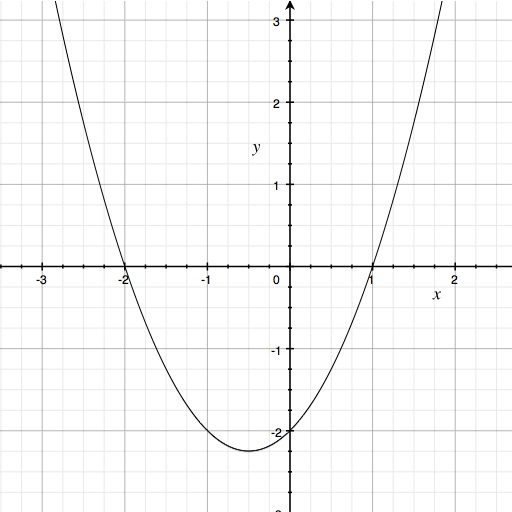## Simple, easy-to-use quadratic equations solver, which uses the quadratic formula for finding the factors of a quadratic equation (of real numbers)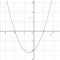by wakachamo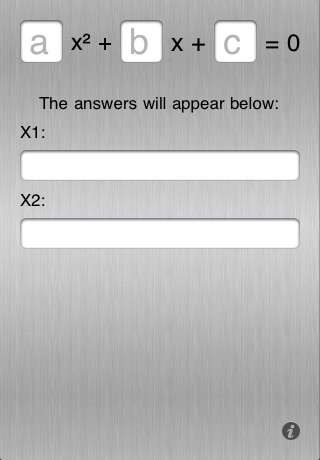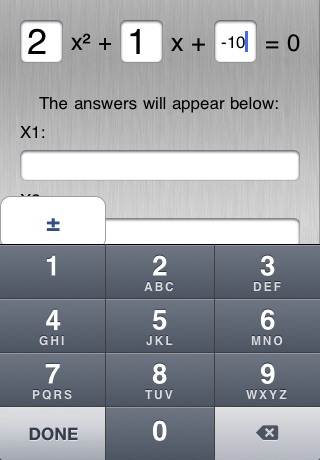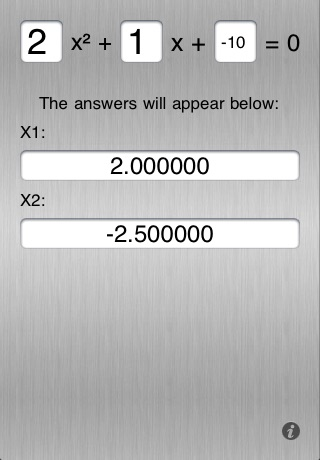Simple, easy-to-use quadratic equations solver, which uses the quadratic formula for finding the factors of a quadratic equation (of real numbers).### App Details

Version
2.0.1
Rating
(3)
Size
0Mb
Genre
Utilities Education
Last updated
January 25, 2011
Release date
December 17, 2009